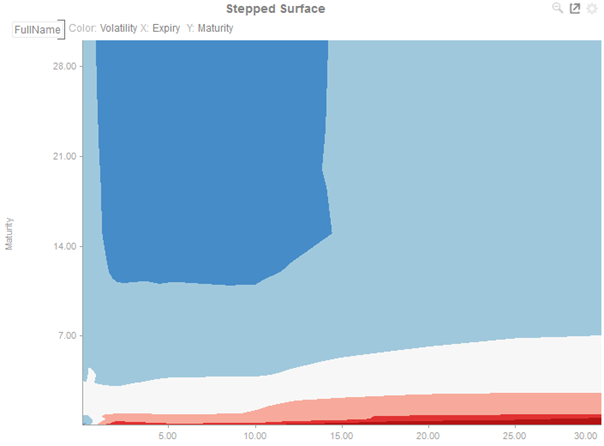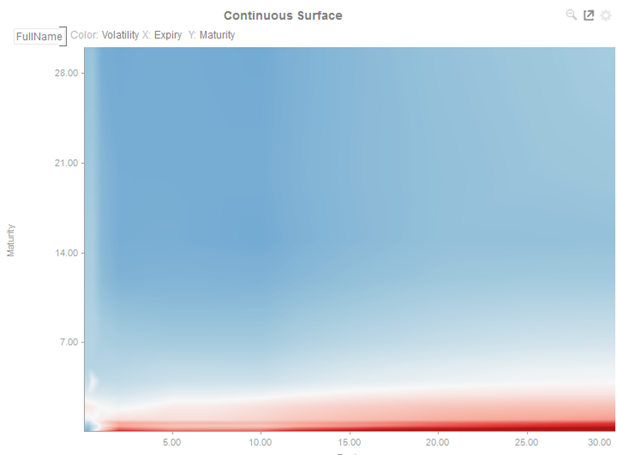Altair® Panopticon

# Surface Plot

Surface Plots are used to identify trends and outliers within numeric surfaces.

The Surface is made up of a series of points where each point has:

• X Position

• Y Position

• Color (represents the Z-axis)

The Surface Plot can support data sets where the X and Y positions can both be regular and irregular in their distribution.

Additionally, the color scale can be continuous or stepped to show a surface gradient.Figure 1. A surface plot with stepped colorsFigure 2. A surface plot with continuous colors